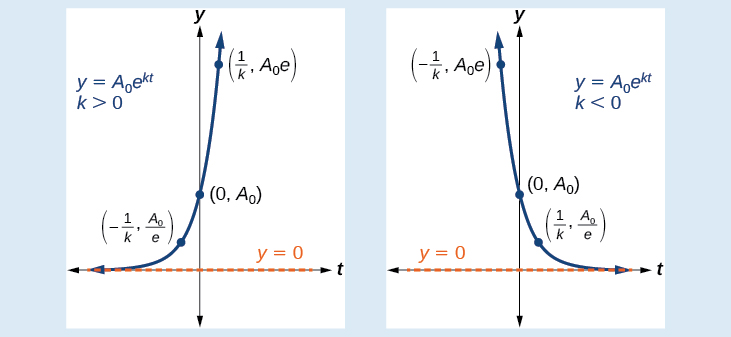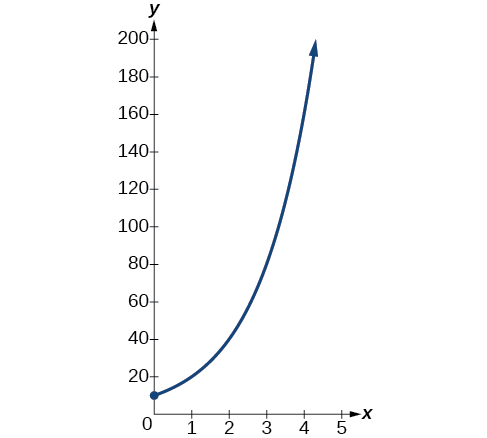6.7 Exponential and logarithmic models  (Page 2/16)

 Page 2 / 16

Characteristics of the exponential function, y = A0e kt

An exponential function with the form $y={A}_{0}{e}^{kt}$ has the following characteristics:

• one-to-one function
• horizontal asymptote: $y=0$
• domain:
• range: $\left(0,\infty \right)$
• x intercept: none
• y-intercept: $\left(0,{A}_{0}\right)$
• increasing if $k>0$ (see [link] )
• decreasing if $k<0$ (see [link] )An exponential function models exponential growth when k > 0 and exponential decay when k < 0.

Graphing exponential growth

A population of bacteria doubles every hour. If the culture started with 10 bacteria, graph the population as a function of time.

When an amount grows at a fixed percent per unit time, the growth is exponential. To find ${A}_{0}$ we use the fact that ${A}_{0}$ is the amount at time zero, so ${A}_{0}=10.$ To find $k,$ use the fact that after one hour $\left(t=1\right)$ the population doubles from $10$ to $20.$ The formula is derived as follows

so $k=\mathrm{ln}\left(2\right).$ Thus the equation we want to graph is $\text{\hspace{0.17em}}y=10{e}^{\left(\mathrm{ln}2\right)t}=10{\left({e}^{\mathrm{ln}2}\right)}^{t}=10·{2}^{t}.\text{\hspace{0.17em}}$ The graph is shown in [link] .The graph of y = 10 e ( ln 2 ) t

Half-life

We now turn to exponential decay . One of the common terms associated with exponential decay, as stated above, is half-life , the length of time it takes an exponentially decaying quantity to decrease to half its original amount. Every radioactive isotope has a half-life, and the process describing the exponential decay of an isotope is called radioactive decay.

To find the half-life of a function describing exponential decay, solve the following equation:

$\frac{1}{2}{A}_{0}={A}_{o}{e}^{kt}$

We find that the half-life depends only on the constant $k$ and not on the starting quantity ${A}_{0}.$

The formula is derived as follows

Since $t,$ the time, is positive, $k$ must, as expected, be negative. This gives us the half-life formula

$t=-\frac{\mathrm{ln}\left(2\right)}{k}$

Given the half-life, find the decay rate.

1. Write $A={A}_{o}{e}^{kt}.$
2. Replace $A$ by $\frac{1}{2}{A}_{0}$ and replace $t$ by the given half-life.
3. Solve to find $k.$ Express $k$ as an exact value (do not round).

Note: It is also possible to find the decay rate using $k=-\frac{\mathrm{ln}\left(2\right)}{t}.$

Finding the function that describes radioactive decay

The half-life of carbon-14 is 5,730 years. Express the amount of carbon-14 remaining as a function of time, $t.$

This formula is derived as follows.

The function that describes this continuous decay is $f\left(t\right)={A}_{0}{e}^{\left(\frac{\mathrm{ln}\left(0.5\right)}{5730}\right)t}.$ We observe that the coefficient of $t,$ $\frac{\mathrm{ln}\left(0.5\right)}{5730}\approx -1.2097×{10}^{-4}$ is negative, as expected in the case of exponential decay.

The half-life of plutonium-244 is 80,000,000 years. Find function gives the amount of carbon-14 remaining as a function of time, measured in years.

$f\left(t\right)={A}_{0}{e}^{-0.0000000087t}$

The formula for radioactive decay is important in radiocarbon dating , which is used to calculate the approximate date a plant or animal died. Radiocarbon dating was discovered in 1949 by Willard Libby, who won a Nobel Prize for his discovery. It compares the difference between the ratio of two isotopes of carbon in an organic artifact or fossil to the ratio of those two isotopes in the air. It is believed to be accurate to within about 1% error for plants or animals that died within the last 60,000 years.

A laser rangefinder is locked on a comet approaching Earth. The distance g(x), in kilometers, of the comet after x days, for x in the interval 0 to 30 days, is given by g(x)=250,000csc(π30x). Graph g(x) on the interval [0, 35]. Evaluate g(5)  and interpret the information. What is the minimum distance between the comet and Earth? When does this occur? To which constant in the equation does this correspond? Find and discuss the meaning of any vertical asymptotes.
The sequence is {1,-1,1-1.....} has
how can we solve this problem
Sin(A+B) = sinBcosA+cosBsinA
Prove it
Eseka
Eseka
hi
Joel
June needs 45 gallons of punch. 2 different coolers. Bigger cooler is 5 times as large as smaller cooler. How many gallons in each cooler?
7.5 and 37.5
Nando
find the sum of 28th term of the AP 3+10+17+---------
I think you should say "28 terms" instead of "28th term"
Vedant
the 28th term is 175
Nando
192
Kenneth
if sequence sn is a such that sn>0 for all n and lim sn=0than prove that lim (s1 s2............ sn) ke hole power n =n
write down the polynomial function with root 1/3,2,-3 with solution
if A and B are subspaces of V prove that (A+B)/B=A/(A-B)
write down the value of each of the following in surd form a)cos(-65°) b)sin(-180°)c)tan(225°)d)tan(135°)
Prove that (sinA/1-cosA - 1-cosA/sinA) (cosA/1-sinA - 1-sinA/cosA) = 4
what is the answer to dividing negative index
In a triangle ABC prove that. (b+c)cosA+(c+a)cosB+(a+b)cisC=a+b+c.
give me the waec 2019 questionsByByByBy